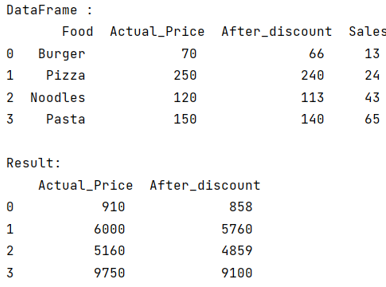# Python - How to multiply columns by a column in Pandas?

Given a DataFrame, we need to multiply columns by a column in this DataFrame. By Pranit Sharma Last updated : September 26, 2023

Pandas is a special tool that allows us to perform complex manipulations of data effectively and efficiently. Inside pandas, we mostly deal with a dataset in the form of DataFrame. DataFrames are 2-dimensional data structures in pandas. DataFrames consist of rows, columns, and data.

## Multiplying columns by a column in Pandas?

To multiply columns by a column, we will first create a DataFrame, the dataframe will contain 2 columns initially and we use pandas.DataFrame.multiply() method and store all the values in another variable. Finally, we will assign this list to a new column of the DataFrame. The DataFrame.multiply() method is used to multiply two dataframe columns, we need to define the column which has to be multiplied inside square brackets with our dataframe like df[col], and then multiply() method will take another column with whom we want to multiply.

Note: If we have multiple columns to multiply, we will pass a list of column names inside square brackets with our dataframe like df[[col1, col2]].

Let us understand with the help of an example,

## Python program to multiply columns by a column in Pandas

```# Importing pandas package
import pandas as pd

# Creating a dictionary
d = {
'Food':['Burger','Pizza','Noodles','Pasta'],
'Actual_Price':[70,250,120,150],
'After_discount':[66,240,113,140],
'Sales':[13,24,43,65]
}

# Creating a dataframe
df = pd.DataFrame(d)

# Display Dataframe
print("DataFrame :\n",df,"\n")

# Multiply columns
result = df[["Actual_Price", "After_discount"]].multiply(df["Sales"], axis="index")

# Display result
print("Result:\n",result)
```

### Output

The output of the above program is: